### How far can a transmitter signal be traced?

This is a question most locator users want to ask, and most manufacturers wish to avoid answering! This is not through deviousness, but simply because there is no way of discovering the answer other than by empirical experiment for any given line, for the reasons discussed earlier. A more practical question is, ‘How can the location distance from a transmitter be increased or maximized?’

The distance over which a signal starting with a given current strength will effectively reduce to zero will depend upon the Rate of Signal Loss for that line. The most distant point at which this signal will still be detectable will be a function of Receiver Sensitivity; for the receiver to detect it, some current must be present, and its signal distinguishable above spurious and interfering signals, termed noise. The higher the current, the easier it is to detect.

The rate of signal loss is a nominally fixed value for a particular line and signal frequency. It depends upon its fundamental electrical characteristics like conductivity, capacitance and inductance relative to ground and other metallic lines or structures, which are in turn related to line diameter, insulation, soil type and conductivity.

The possibilities for increasing detection distances are therefore:

1. Reduce the rate of signal loss
2. Increase the signal current

Let us examine each of these in turn. First it is necessary to appreciate the necessity of using a logarithmic or decibel scale for any graph of rate of signal loss along a line, if meaningful information is to be conveyed.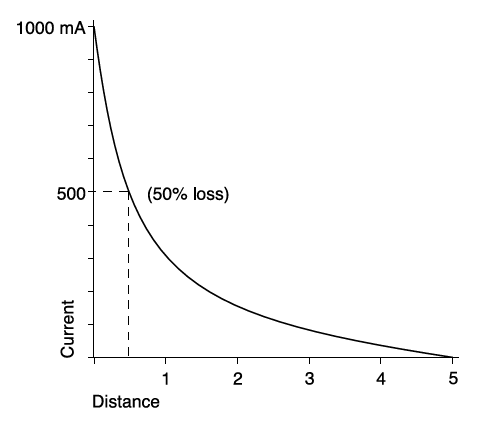The graph illustrates the way a signal current of 1A decays along a given line. It is clear that most of the signal is lost in the line nearest the transmitter, after which there is a more gradual slope down from a low value to zero.

It is in this gradual slope at low values that we are interested, where small changes in level make significant changes in distance, so it is therefore necessary to expand this part of the curve. This is done by changing the vertical axis to a logarithmic scale, i.e. by substituting its logarithm for the original value. Decibels are simply standard logarithms multiplied by 20 for convenience, and express the ratio between levels. For example, an increase of 6dB represents a doubling, a decrease of 6dB a halving of level. The effect is to change the curve to a straight line, making level comparisons more visually obvious.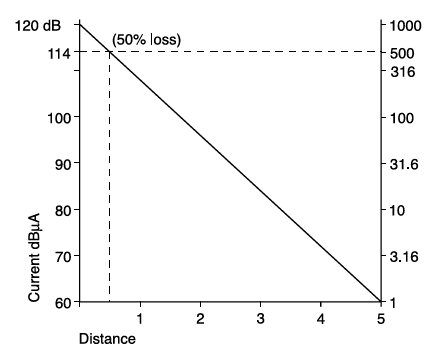Reducing the rate of signal loss

Clearly it is not possible to alter the characteristic properties of the line itself. However, one can try and tailor the transmitter signal to give it the best chance of travelling furthest, and this is where choice of signal frequency is important. Generally, a lower frequency will travel further than a higher, because the latter leaks away quicker via capacitance to ground. In the graph below, the distances at which the same signal current will have fallen to 0 dB are represented by D33, D8 and D1 for 33kHz, 8kHz and 512Hz signals respectively.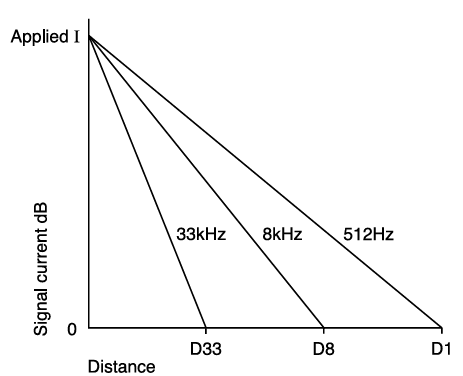Note, however, that this is by no means the only consideration. Which frequency can be applied most effectively will vary with the means, e.g., higher frequencies are much easier to apply by induction.

The background noise level will also differ for different frequencies, particularly within the power frequency harmonic range, and receiver sensitivities may also be affected. There is likely to be an optimum frequency band for any particular line and situation, best established by experiment.

### Increasing the signal current

There are basically three ways of achieving this, costing nothing, a little, and a lot respectively! The one which costs nothing seems obvious, but is often forgotten - make sure there is the best possible ground connection for return of the signal.

The second, which costs a little in equipment design, is to use impedance matching. Any given line will have a fixed impedance at any particular frequency, but its detection will require a certain signal current flow. The voltage required to drive that current through the line will be the product of that current and line impedance seen from the transmitter. For instance, a line impedance of 10 Ohm would require 10V per amp, while an impedance of 200 Ohm would need 200V per amp. If the transmitter is only capable of delivering its full output at a fixed voltage, say 50V, it could drive 5A through the first line, but only 0.25A through the second. So the power transferred into the second line would be much lower than the first (250VA and 12.5VA respectively) while the low impedance line might overload the transmitter at full output. To optimize the transmitter capability, it is clearly desirable to make its output available at more than one voltage/current combination. It is analogous to gear changing, in that the ideal would be an infinitely variable ratio, but practicalities mean that step changes are more economic. Just having two choices gives a considerable advantage over a single voltage capability without increasing the power required.

The third, but costliest, method of increasing current is to increase the transmitter power. Note that a square law applies here, so that in order to double the signal current, a transmitter at four times the power is needed. That not only increases the size, weight and cost of the transmitter elements; it increases the battery drain for portable locators, which then either have a shorter operating time between replacement or recharging, or are larger, heavier, and more costly.

Apart from these practical disadvantages, there is also a safety implication. As we have seen under impedance matching, a high-impedance line may need a high transmitter voltage to impose a significant current on it, and this may take it outside the limits of safe use.

Whatever means is adopted, the effect of increasing signal current with a given line and frequency will be to increase distance. The effect of doubling the transmitter current is typified in the graph below, in which the 0dB current level point distance increases from D1 to D2.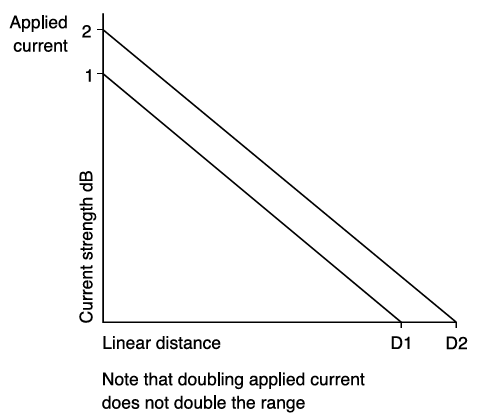The graphs so far have shown the way signal current falls to virtually zero along a line. But long before this point, the signal current level usually becomes undetectable by the receiver, because it is indistinguishable from background noise and interference levels. It is this noise threshold which is the significant figure to be considered; the graph below repeats the comparison of rate of signal loss for different frequencies, but this time shows the effect of different noise thresholds. While the 0dB distance D1 for 512Hz is significantly greater than D8 for 8kHz, the receiver noise threshold T1 at 512Hz is higher than T8 at 8kHz, because it falls within the harmonic frequencies associated with 50 and 60Hz power systems, which is present in strength in most working environments. So the maximum detection distance R1 for 512Hz is not as much further than R8 for 8kHz as the original comparison might suggest. A similar effect is apparent in comparing the 33 and 8kHz detection distances.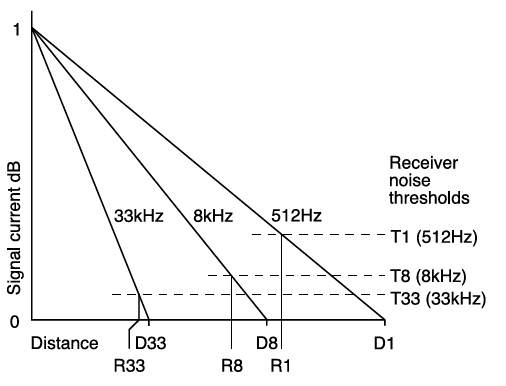It is therefore apparent that receiver sensitivity is an extremely important factor in detection range. Achieving a low noise threshold is not only a function of high amplification or gain. The quality of filtering, i.e. rejection of signals at frequencies other than the transmitter signal frequency, is a vital element. The graph below repeats the comparison of doubling signal current, but this time shows that the same increase of detection distance from R1 to R2 is achievable by halving the receiver noise threshold from T1 to T2 as by doubling the signal current.

### Summary

The ultimate limit of detection range for any given line and signal frequency is set by receiver sensitivity, which is a function as much of filter quality as amplification capability and aerial design.

How distant this limiting point is from the transmitter will vary with the line, the frequency, and also with the ability of the transmitter to impose a high signal current on it. This is a function not only of power but of impedance matching, as well as making sure of a good ground return path.

Transmitter power requirements rise as the square of signal current, so impose cost, weight and battery life penalties, as well as the prospect of unacceptably high connection voltages for general usage if high currents are to be applied to high impedance lines.

Cost-effective range optimization is therefore achieved by the choice of the signal frequency, transmitter current/ voltage options within battery life and operator safety limits, and above all in the quality of the receiver system in terms of dynamic range, that is to say the ratio of maximum to minimum signal level which it will process.